## Electric Field

An electric field is said to exist at a point if a force of electrical origin is exerted on a stationary charged body placed at that point. Quantitatively, the electric field or electric intensity or the electric field strength at a point is defined as the force experienced by a unit positive test charge placed at that point, without disturbing the position of source charge.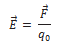The electric field at a point is defined as the electrostatic force per unit test charge acting on a vanishingly small positive test charge placed at that point. Hence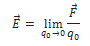The electric field is a vector quantity whose direction is same as that of the force exerted on a positive test charge.

SI unit is Newton per coulomb (NC-1). It is equivalent to volt per meter (Vm-1).

Dimension of Electric field is [MLT-3A-1].

## Electric Field Due to a Point Charge

A single point charge has the simplest electric field.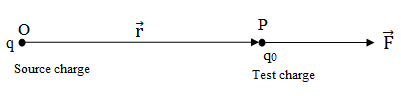According to Coulomb’s law, the force on charge q0 is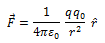Electric field at point P is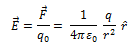The magnitude of the field isClearly, E ∝ 1/r2. This means that at all points on the spherical surface drawn around the point charge, the magnitude of is same and does not depend on the direction of . such a field is called spherically symmetric or radial field, i.e., a field which looks the same in all direction when seen from the point charge.

## Electric Field due to a System of Charges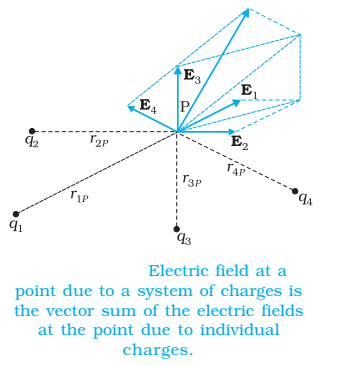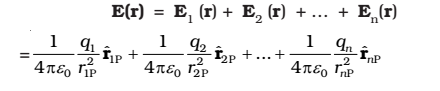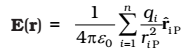E is a vector quantity that varies from one point to another point in space and is determined from the positions of the source charges.